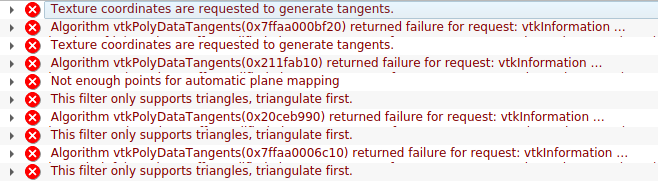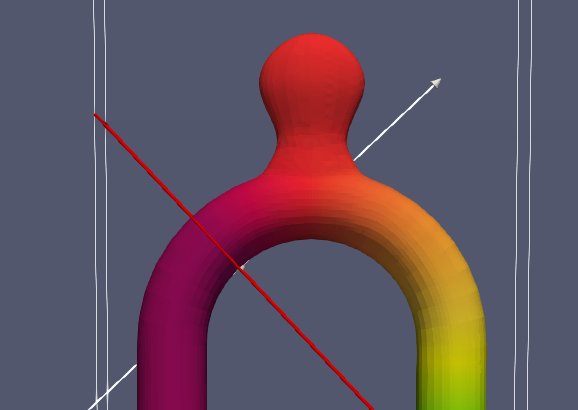# Calculation of surface tangents error

Hello,

I want to apply the filter “Generate Surface Tangents” on a surface and I get the following error (attached picture)The filter “Generate Surface Nomrlas” works fine. What I have to do for “Generate Surface Tangents” filter?

Thanks

Thanks for the reply. Do you mean to share pictures or data?

Here you can download the Data. Actually I want to calculate the normal and the tangent vectors on the wall surface of my geometry.

I just requested access

I gave you access!

Your data does not have any TCoords, so tangents vectors cant be computed.

What are you trying to achieve ?

What are TCoords? I want to take slices on the cross section like thisTo achieve that I have to know the tangent vector on the surface point (like this in picture) in order to make a slice paralllel to each cross section of the curved part. Then I want to take the dot product of the velocity vector with the normal vector on the surface point in order to calculate the flow on this plane (slice).

Just compute that with a calculator

Yes I know that but my problem is how to produce slices parallel to each cross section like this in the picture? That is my problem, because the slice has to have an arbitrary direction to be parallel to the cross section. This direction is the tangent vector of a point on the outer slice surface

Just compute what you need with a calculator.

I don’t quite get the issue here. A tangent at a surface for a given point is a plane. The only thing you need to fully define a plane is a normal vector and a point. If your data doesn’t have a normal then you can just create one using the `Generate Surface Normals` filter.

What behavior are you expecting from the `Generate Surface Tangent` exactly ?

So lets make it clear.

I have the following geometryI want to make slices on the curved part. To do this I need a point P (ok this is easy) and a normal vector V_s to define the plane. For the slice to be parallel to the cross section like this in the first picture, we have to know the tangent vector on the point P we selected. In other case we will have something like that

which is wrong because the slice does not follow the arc properly. The normal vector V_s is the tangent vector on point P like this

So my question is how to know the vector V_s.

Actually there is an infinity of tangent vector at point P, but I get what you want. What you really want is the tangent of the center of your geometry (ie the red line on your schema), because the tangent of a line is a vector and not a plane. There is no way of computing this “center of geometry” line using existing ParaView filters AFAIK so as Mathieu said, you can just compute what you want manually using the python calculator.

Also note that although it’s easy to create multiple slices that have the same normal, it’s not trivial when they’re not the same, as it is in your case. You’d have to create a slice filter for each slice with a different normal.

In the end: there’s no existing filters or smart workaround for your use case AFAIK. You can always try to hack your way around by using the python calculator or by creating your own plugin.

Thank you very much for your answer. Yes I understand that there is an infinity of tangent vectors but I thought that paraview could handle this issue. I will make a custom code with python. Thanks again

1 Like

No problemside note : you can also take a look at the `Slice Along Polyline` filter, it could be usefull for your use case